## What's our fair?¶

First, let's take a good look at our data.

In :
import numpy as np
import pandas as pd
from matplotlib import pyplot as plt

In :
plt.rcParams['figure.figsize'] = (8.0, 6.0)
plt.rc('font', size=16)


We have 10 years of historical data from 2010-2019 of electricity consumption and prices

In :
!ls case1/DATA

2010.csv  2012.csv  2014.csv  2016.csv	2018.csv
2011.csv  2013.csv  2015.csv  2017.csv	2019.csv

In :
data = [pd.read_csv(f'case1/DATA/{year}.csv', header=0, names=['date', 'cons', 'p']) for year in range(2010, 2020)]

In :
data.head()

Out:
date cons p
0 2010-01-01 0.000000 0.0
1 2010-01-02 0.447285 2.0
2 2010-01-03 14.339777 2.0
3 2010-01-04 17.388527 2.0
4 2010-01-05 28.372350 2.0
In :
plt.xlabel("Day")
plt.ylabel("Cumulative Consumption (KWh)")
plt.title("Consumption/day in 2010")
plt.plot(data.cons)
plt.show()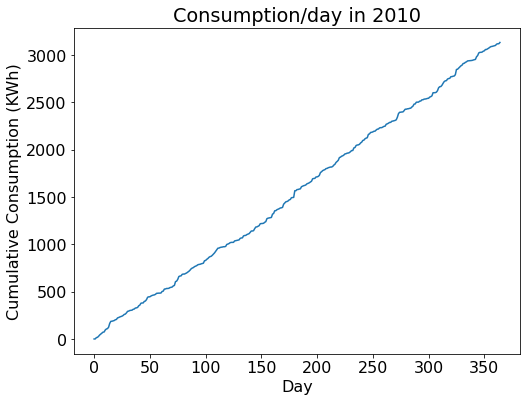#### Price/day in 2010¶

The 4 contract expiry dates are plotted as vertical red lines.

In :
plt.xlabel("Day")
plt.ylabel("Price ($)") plt.ylim(0, 4.8) plt.axvline(180, c="red") plt.axvline(242, c="red") plt.axvline(302, c="red") plt.axvline(364, c="red") plt.plot(data.p[1:]) plt.show()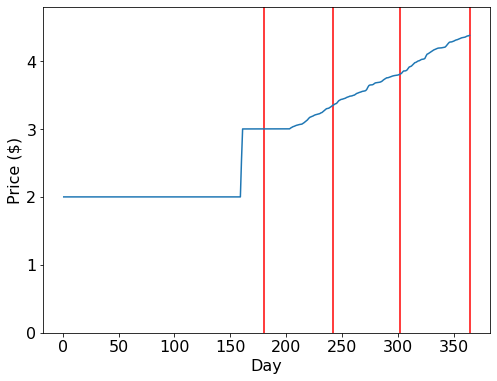In : plt.title("Daily consumption in 2010") plt.xlabel("Daily consumption (KWh)") plt.ylabel("Frequency") plt.hist(data.cons[1:].values - data.cons[:-1].values, 50) plt.show()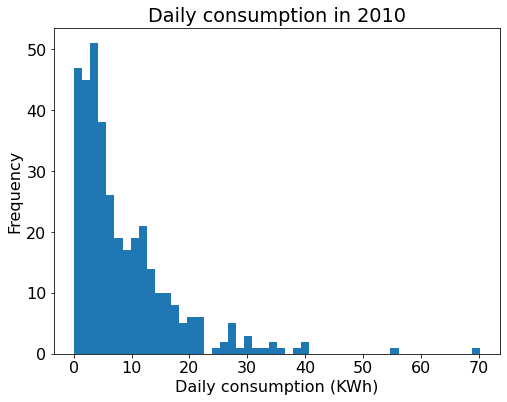In : # Convert cumulative consumption to daily for d in data: d['dailycons'] = d.cons - d.cons.shift(1)  ### Gas and coal supplies¶ All 10 years have very similar consumption/ production patterns, so we should be able to model the electricity price accurately. We will investigate the pattern of gas/coal consumption over the 10 years. In : gas = [] for year in data: row = year[(year.p < 3) & (year.p > 2)] gas.append((row.cons - (3 - row.p) * row.dailycons).values) plt.title("Gas capacity/Year") plt.xlabel("Year") plt.ylabel("Gas capacity (KWh)") plt.plot(range(2010, 2020), gas) plt.show()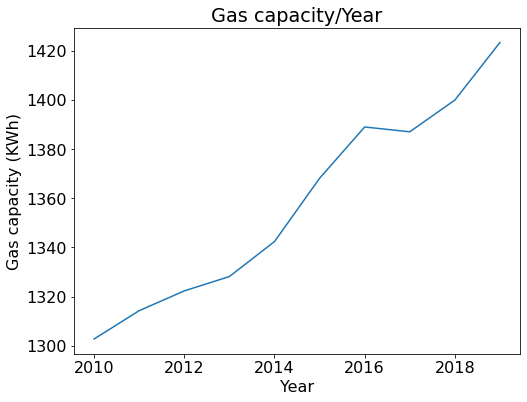In : coal = [] for i, year in enumerate(data): row = year[(year.p < 3.01) & (year.p > 3)].iloc c = row.cons - (row.p - 3) * 1000 - gas[i] coal.append(c) plt.title("Coal capacity/Year") plt.xlabel("Year") plt.ylabel("Coal capacity (KWh)") plt.plot(range(2010, 2020), coal) plt.show()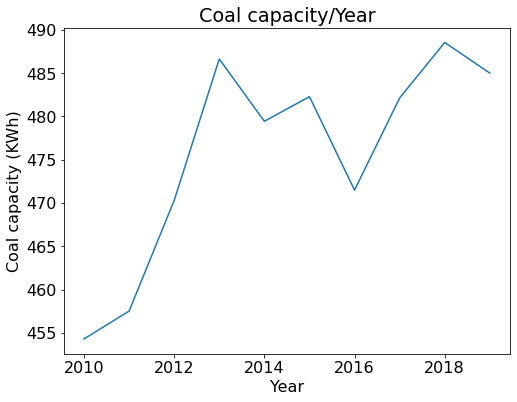It looks like there is a linear growth in natural gas and coal capacity over the years. Perhaps we can model them with linear models. In : from sklearn.linear_model import LinearRegression  In : gasreg = LinearRegression().fit(np.arange(10).reshape(-1, 1), gas) print("Gas production") print("m:", gasreg.coef_, "c:", gasreg.intercept_) print("2020 mean:", gasreg.predict([])) print("Unbiased estimate of variance:", np.var(gasreg.predict(np.arange(10).reshape(-1, 1)) - gas) * 10/9)  Gas production m: 13.419965801206784 c: 1297.330695128393 2020 mean: 1431.5303531404609 Unbiased estimate of variance: 45.99518922123725  In : coalreg = LinearRegression().fit(np.arange(10).reshape(-1, 1), coal) print("Coal production") print("m:", coalreg.coef_, "c:", coalreg.intercept_) print("2020 mean:", coalreg.predict([])) print("Unbiased estimate of variance:", np.var(coalreg.predict(np.arange(10).reshape(-1, 1)) - coal) * 10/9)  Coal production m: 3.0905406916305362 c: 461.8418539140977 2020 mean: 492.7472608304031 Unbiased estimate of variance: 57.38547189035349  ### Modelling Consumption¶ Once we have a good idea of gas/coal capacity, we have to investigate consumption patterns. Specifically, we want to answer the following questions: • What's the underlying distribution of consumption? • Is the consumption distribution constant or time-varying? (Periodic?) • Are there any autocorrelations in the consumption? (Perhaps, high consumption predicts high consumption the next day?) In : # Combining consumption over 10 years cons = pd.concat([year.dailycons[1:] for year in data]).reset_index().drop('index', axis=1).values.T  In : plt.title("Consumption/Day") plt.xlabel("Day") plt.ylabel("Consumption (KWh)") plt.plot(cons) plt.show()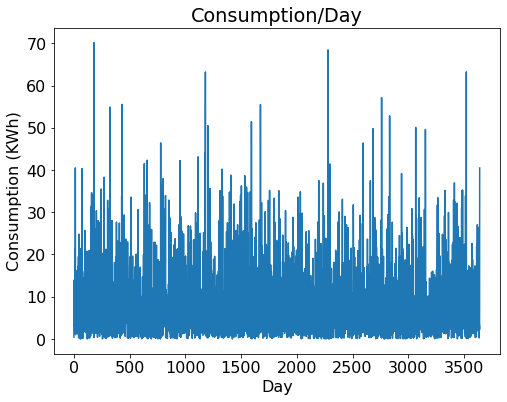In : plt.title("Histogram of consumption") plt.xlabel("Consumption (KWh)") plt.ylabel("Frequency") plt.hist(cons, 100) plt.show()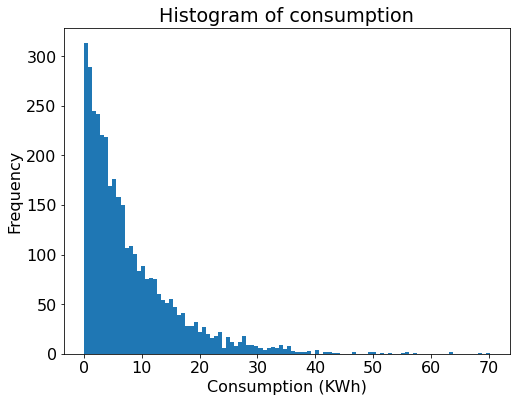#### Hmm, does this look like an exponential distribution?¶ The exponential distribution has PDF of$\lambda e^{-\lambda x}$, with mean of$\frac{1}{\lambda}$In : lmb = 1 / np.mean(cons) print(lmb)  0.1252364948706893  In : plt.title("Histogram of consumption") plt.xlabel("Consumption (KWh)") plt.ylabel("Frequency") plt.hist(cons, range(0, 72)) plt.plot(np.linspace(0, 70, 100), len(cons) * lmb * np.exp(-lmb * np.linspace(0, 70, 100)), label="Exp(lmb)") plt.legend(loc="upper right") plt.show()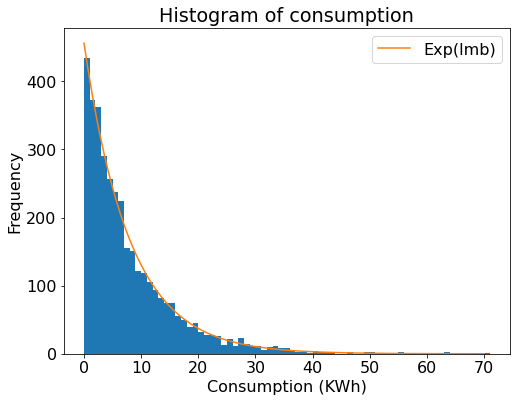In : print("Sample variance:", np.var(cons)) print("Exp distribution variance:", 1/lmb**2)  Sample variance: 66.954336216657 Exp distribution variance: 63.758514788476  We have a strong suspicion that the underlying distribution is exponential. But we still have to confirm that there aren't any periodic patterns in the data. #### Autocorrlelation between consecutive days.¶ The low correlation suggests lack of relationship between consecutive days. In : np.corrcoef(cons[1:], cons[:-1])  Out: array([[ 1. , -0.02293147], [-0.02293147, 1. ]]) #### Exponentially weighted moving averages of consumption.¶ There doesn't seem to be a clear periodic trend in consumptions In : plt.title("EWMA of consumption, alpha = .01") plt.xlabel("Day") plt.ylabel("EWMA(cons)") plt.plot(pd.Series(cons).ewm(alpha=.01).mean()) plt.show() plt.title("EWMA of consumption, alpha = .005") plt.xlabel("Day") plt.ylabel("EWMA(cons)") plt.plot(pd.Series(cons).ewm(alpha=.005).mean()) plt.show()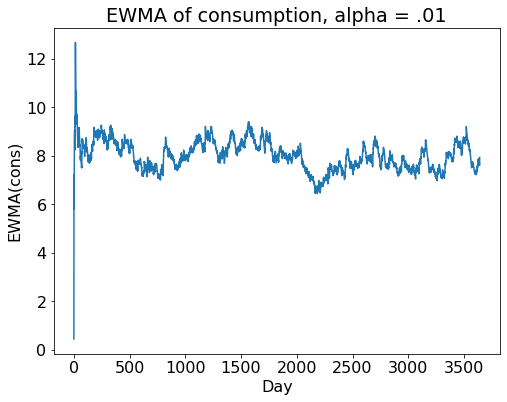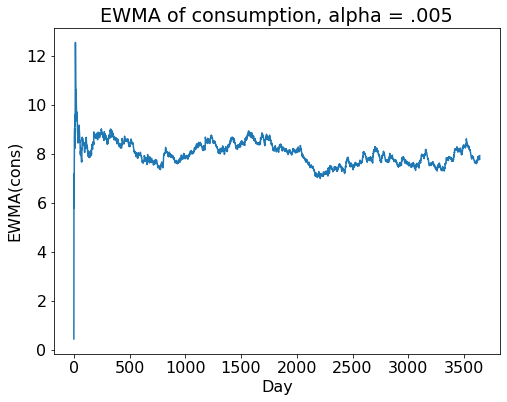#### Correlations for n-day periods, n$\in$[1, 50)¶ Again, we fail to observe any interesting patterns In : for i in range(1, 50): corr = np.corrcoef(cons[i:], cons[:-i]) if abs(corr) > .03: print(i, corr)  10 0.045732008518092615 14 -0.03654910372937772 19 -0.03024981752334226 24 -0.03749542551664027 43 0.03193238383270123 47 -0.031447572727001556  ## Putting it all together¶ Now that we're satisfied with modelling consumption as an exponential distribution, we can predict the price of the futures contracts. We begin by looking at past contract prices. In : print("\t".join(["Year", "June", "Aug", "Oct", "Dec"])) for year in data: days = np.array([180, 242, 302, 364]) if int(year.loc.date[:4]) % 4 == 0: # Leap year days += 1 print(year.loc.date[:4], *np.round(year.loc[days].p.values, 2), sep="\t")  Year June Aug Oct Dec 2010 3.0 3.35 3.81 4.38 2011 3.0 3.12 3.58 4.01 2012 3.0 3.15 3.64 4.21 2013 3.0 3.2 3.72 4.23 2014 3.0 3.32 3.85 4.25 2015 3.0 3.05 3.44 3.8 2016 2.0 3.0 3.48 3.95 2017 3.0 3.09 3.55 3.96 2018 2.0 3.09 3.44 3.91 2019 3.0 3.15 3.54 4.02  #### August, October and December contracts¶ We'll ignore the June contract for now (it's a little more mathematically complicated to price). Historically, these 3 contracts expire only after natural gas capacity is used up, and therefore their prices would be \$3.00 + \$.001 * amount of electricity usage exceeding coal capacity. Let N be the number of days until the contract expires, G be the expected natural gas capacity (KWh), and C be the expected coal capacity (KWh), E be the amount of electricity already consumed (KWh). Then, the expected value of the contract is$3.00 + max(0, .001 \times (\frac{1}{\lambda} \times N + E - G - C))$, where$\lambda$= 0.1252364948706893 was computed earlier. Here's our predictive formula in action for the December contract in 2015. In : G = gasreg.predict(np.full((1,1), 5)) C = coalreg.predict(np.full((1,1), 5)) print(f'G = {G:.2f}, C = {C:.2f}') data["pred"] = 3.00 + .001 * (data.cons + 1/lmb * np.arange(364, -1, -1) - G - C) plt.ylim(3.1, 4.3) plt.plot(data.pred, label="Predicted price") plt.axhline(3.80, c="red", label="True price") plt.plot(np.arange(75, 365), pd.Series(data.dailycons).ewm(alpha=.001).mean()[75:] / 2.3, label="EWMA(Consumption), scaled") plt.legend(loc="lower left") plt.xlabel("Day in 2015") plt.ylabel("Price ($)")

plt.show()

G = 1364.43, C = 477.29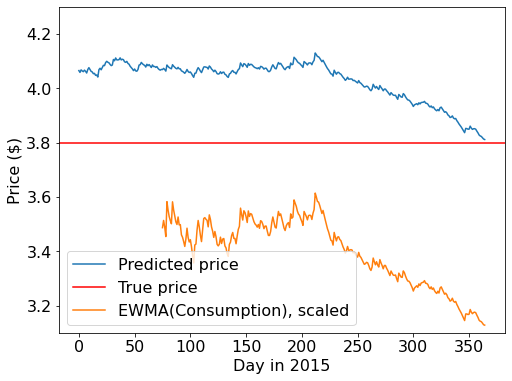As the diagram shows, the model overestimates the contract price initially, but approached the true value closer to the contract expiry date. This is mainly because consumption decreased significantly over the later half of the year, bringing down the final electricity price. This seemed good enough for our purposes.

#### A small improvement in G and C predictions¶

We used the mean value of the regression prediction for gas and coal capacity in the previous example, but it could result in ridiculous situations where the predicted capacity is lower than the capacity we have already observed. If we predicted 1300KWh of gas capacity but have already observed 1320KWh of gas being used, then we should adjust our expectations for the amount of total gas capacity.

Since we assumed a normal distribution for gas capacity, N($\mu$, $\sigma^2$), we can change it to a truncated normal distribution by cutting off the impossible lower tail. This is basically combining a normal prior with an uniform posterior starting from the observed capacity.

The June contract is different from the other contracts. Historically, it either settled to \$2 or \$3, depending on whether natural gas capacity was exhausted by expiry, with 2 of 10 cases expiring at \$2. Our previous approach would not be able to accurately price the June contract at all! There are 2 ways around this: using the Central Limit Theorem (CLT) to assume normality in the distribution of consumption, or to use the Erlang distribution to compute the probability exactly using marginalization and integration. We went with the latter, but I'll show both methods and the differences in their predictions. #### Assuming normality using CLT¶ From 10 years of consumption data, we have mean consumption of 7.98 and variance of 66.95. If there are N days till the expiry of the June contract (and N sufficiently large), and X is the amount of electricity consumed during the N days, then by the CLT X ~ N(7.98 N, 66.95 N). On the other hand, remaining gas capacity G ~ N($\mu$,$\sigma^2$). G - X ~ N($\mu$- 7.98N,$\sigma^2$+ 66.95N) Probability of settling at \$3, P = P(G-X < 0) = norm.cdf(0, loc=$\mu$ - 7.98N, scale=$\sigma^2$ + 66.95N)

Fair price of June contract = $2 + P We demonstrate this with 2016 data, which interestingly settled at$2.

In :
from scipy.stats import norm

In :
G = gasreg.predict(np.full((1,1), 6))
mu = G - data.cons[:181] - 7.98 * np.arange(181, 0, -1)
std = np.sqrt(46 + 66.95 * np.arange(181, 0, -1))
P = norm.cdf(0, loc=mu, scale=std)
price = 2 + P

plt.plot(price, label="Predicted price")
plt.ylim(2, 3)
plt.xlabel("Day in 2016")
plt.ylabel("Price")
plt.show()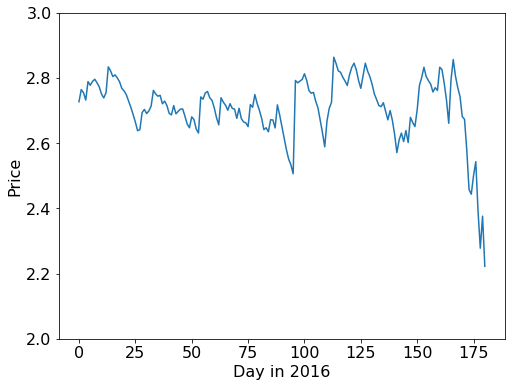#### On the Erlang Distribution¶

Suppose there are N days until the contract expiry. The amount of electricity used in one day ~ Exp($\lambda$). What's the distribution of the amount of electricity, E, used in N days?

It turns out that E is an Erlang distribution with k = N and rate = $\lambda$. If you want to learn more about the derivation, you can read this resource.

Here's what the distribution looks like for various values of N, compared to the normal distribution. For large values for N, the normal distribution closely approximates the Erlang distribution, but at smaller values of N the difference diverges significantly, motivating us to compute the probabilities more accurately.

In :
for n in [1, 5, 20, 50]:
plt.title(f"N = {n}")
x = np.linspace(-n/lmb, n / lmb * 3, 100)
plt.xlabel("x")
plt.ylabel("P(X=x)")
plt.plot(x, gamma.pdf(x, n, scale=1/lmb), label=f"Erlang(n, {1/lmb:.2f})")
plt.plot(x, norm.pdf(x, loc=n/lmb, scale=np.sqrt(n)/lmb), label=f"N({n/lmb:.2f}, {np.sqrt(n)/lmb:.2f})")
plt.show()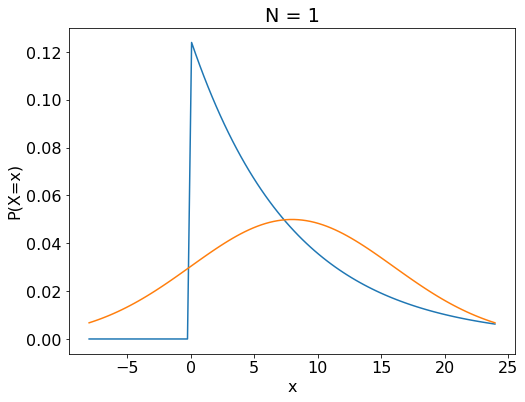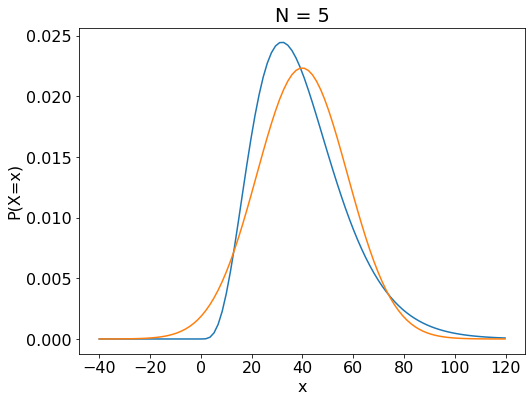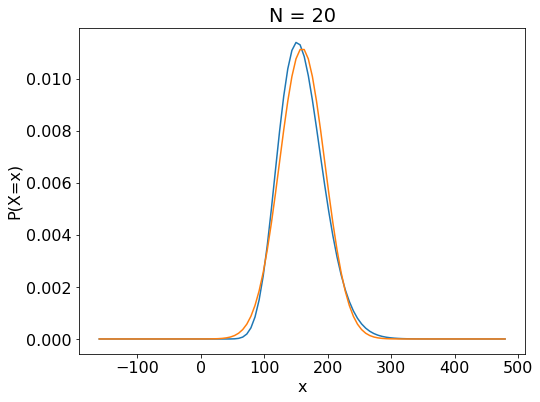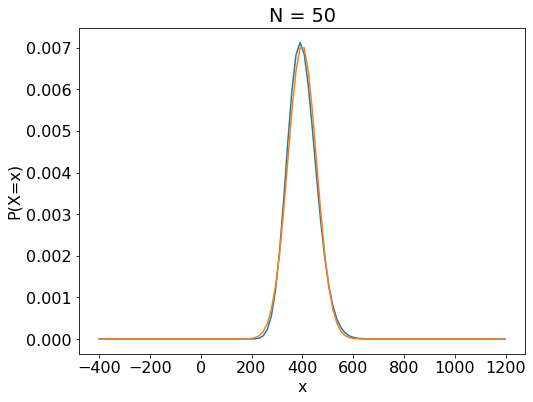#### Direct computation using the Erlang Distribution¶

Once again, we model the remaining gas G ~ N($\mu$, $\sigma^2$).

We want to know P = P(G < E) = P(G - E < 0).

P(G - E < 0) = $\int_{G} P(g - E < 0) P(G = g) dG$

While this integral looks daunting to compute mathematically, we can simply compute it numerically to enough precision. We reduce the infinite range (-$\inf$, $\inf$) to ($\mu$ - 5$\sigma$, $\mu$ + 5$\sigma$), and take k = 10000 evenly spaced points in this range. With this, our integral becomes a summation which we can compute via a dot product.

P(G - E < 0) = $\sum_{\mu - 5\sigma}^{\mu + 5\sigma} P(g - E < 0) P(G = g)$

In :
from scipy.stats import gamma

In :
G = gasreg.predict(np.full((1,1), 6))
def prob_is_gas2(gas_left, n_days):
gas_var = 66.95
n_pts = 10000
x_range = np.linspace(-5*(gas_var**0.5), min(5*(gas_var**0.5), gas_left), n_pts)
nms = norm.pdf(x_range, scale=gas_var**0.5)
gams = gamma.cdf(gas_left - x_range, n_days, scale=1/lmb)
ans = np.dot(gams,nms)
ans /= np.sum(nms)
return ans

direct_pred = 3 - data[:181].apply(lambda row: prob_is_gas2(G - row.cons, 181 - row.name), axis=1)

plt.plot(price, label="CLT pred")
plt.plot(direct_pred, label="Direct pred")
plt.ylim(2, 3)
plt.xlabel("Day in 2016")
plt.ylabel("Price")
plt.legend(loc="lower left")
plt.show()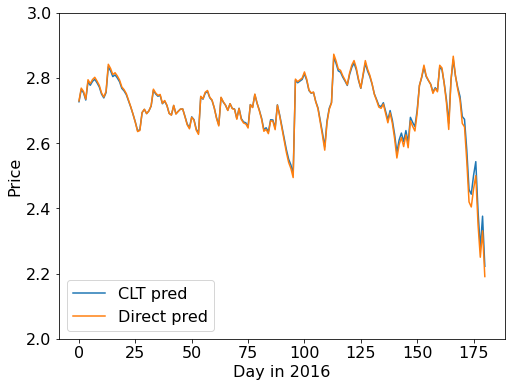The CLT prediction seems to be good enough for most purposes. Zooming into the last few days, we see that the prices diverge by a few cents, still significant but in our competition's context isn't going to make a big difference in profitability.

In :
plt.plot(price, label="Normal pred")
plt.plot(direct_pred, label="Direct pred")
plt.ylim(2, 3)
plt.xlim(160, 182)
plt.xlabel("Day in 2016")
plt.ylabel("Price")
plt.legend(loc="lower left")
plt.show()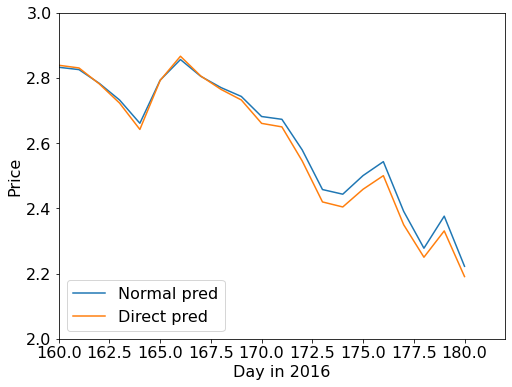#### Fairs = computed¶

Before the competition, we put a lot of effort into analyzing historical data and computing our fairs, so we thought that a lot of our success came from these good fairs. Looking back, these fairs may have put us above the poor or middle performing teams, but didn't differentiate us from the other top teams. Read on for what really mattered!